Geometry

# Circles - Central Angles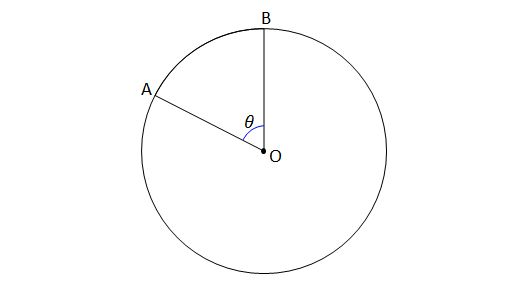In the above diagram, if the radius of the circle is $18$ and the angle $AOB$ (central angle) is ${30}^\circ,$ what is the measure of the arc $\widehat{AB} ?$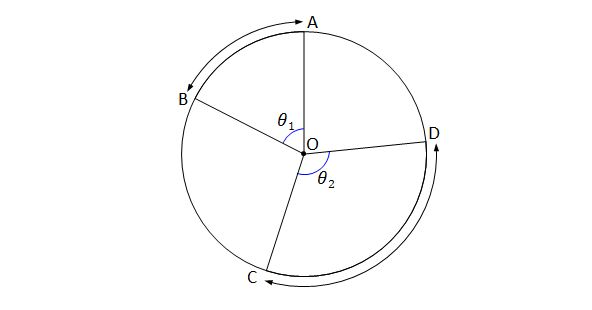In the above diagram, if angle $COD$ is $\theta_2={123}^\circ$ and the measures of arc $\widehat{AB}$ and arc $\widehat{CD}$ are $5\text{ cm}$ and $15\text{ cm},$ respectively, what is the angle $\theta_1 (= \angle AOB)?$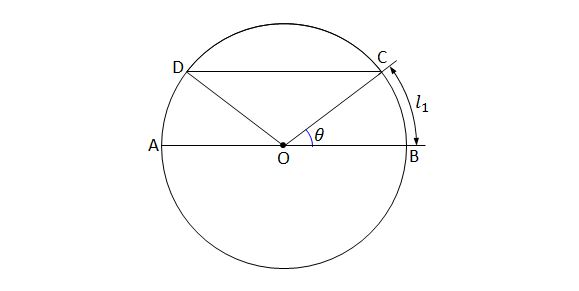In the above diagram, $\overline{AB}$ is a diameter of the circle centered at $O$, $\overline{AB} \parallel \overline{DC}$, and $\angle BOC=\theta={30}^\circ.$ If the measure of the arc $\widehat{BC}$ is $l_1=12\pi\text{ cm},$ what is the measure of the arc $\widehat{CD} ?$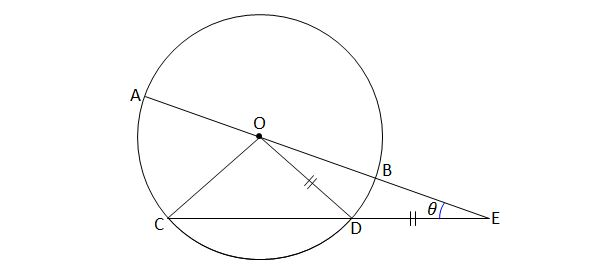In the above diagram, $\overline{AB}$ is a diameter of the circle centered at $O,$ and $\lvert{\overline{OD}}\rvert=\lvert{\overline{DE}}\rvert$ and $\angle E=\theta={15}^\circ.$ If the measure of the arc $\widehat{BD}$ is $5\text{ cm},$ what is the measure of the arc $\widehat{AC}$ in $\text{cm} ?$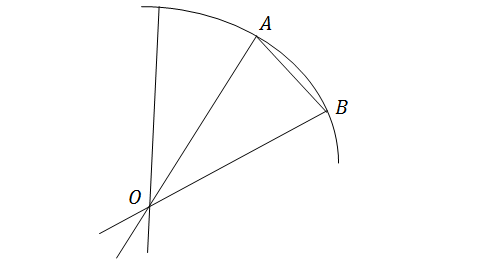Imagine a circle divided into equal sectors via $n$ straight lines that cross point $O$, the center of the circle. Let sector $ABO$ be one of those sectors. When $n=20,$ what is $\angle OAB$ in degrees? (Round down to the nearest degree if there is a decimal.)

×